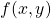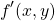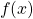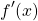# The London Mathematical Society

In November '18, I decided to join the London Mathematical Society (LMS). Last month I attended a meeting there in Russell Square in Central London which involved a couple of lectures and a "swearing in" of new members.

As a part of the swearing in, new members have the opportunity to sign the LMS Member's Book. Interesting thing about this is it dates back to 1865(!). So after signing it, I flicked back the pages to find the signatures of both Arthur Cayley on June 19th 1865:

and James Clerk Maxwell on April 25th 1867:

Study has been so busy this year, I've failed in keeping an up-to-date account of how it's been going. Here's a very quick run-though:

Assignment 2:

• First and second-order differential equations.
• Vector algebra and statics. (There are two blocks connected with taut string on a ramp and nothing is moving, what are the forces?)
• Dynamics. (A block is sliding down a ramp with a certain friction, what are the forces?)

Assignment 3:

• Matrices and determinants.
• Eigenvalues and eigenvectors.
• Systems of linear differential equations. (solving simultaneous differential equations).
• Functions of several variables. (andand).

Assignment 4:

• Mathematical modelling. (Introduction to writing a mathematical modelling paper).
• Oscillations and energy. (Forces in a spring system, and potential energy).
• Forcing, damping an resonance. (Forces in a system of springs and dampers).
• Normal modes. (Oscillations of particles in a spring system).

Assignment 5:

• Systems of differential equations. (Equilibrium points of two differential equations and use of the Jacobian matrix).
• Fourier series.
• Partial differential equations.

Assignment 6:

• Vector calculus. (Scalar fields and gradients).
• Further vector calculus. (Conservative vector fields, curl, and divergence).
• Multiple integrals. (Area and volume integrals).

Assignment 7:

• Writing a 3000-word mathematical modelling paper.

All the above are now complete, and I'm currently working through the last few units. Namely:

• Systems of particles.
• Circular motion.
• Rotating bodies and angular momentum.

There's a requirement for me to submit one assignment per month, from October to May. These double-credit modules are hectic...

Out of all of the above, Assignment 7 had to be the most nerve-racking. Most assignments normally read like exam papers, though for Assignment 7 I had to write a report. So not only had I never written a report like this on this course before, I'd never written a report like this ever! Results are due in a couple of weeks, so based on the outcome I'll be keen to break down where I went wrong.

Favourite bits from the above have to include my first ever hand-calculation of a Fourier series in Assignment 5, and a question where I had to prove a result of Archimedes (287-212 BCE) regarding relative volumes, using modern calculus in Assignment 6. I'd also always wanted to know more about the construction of a Jacobian, so Assignment 5 was good for applying my new knowledge.